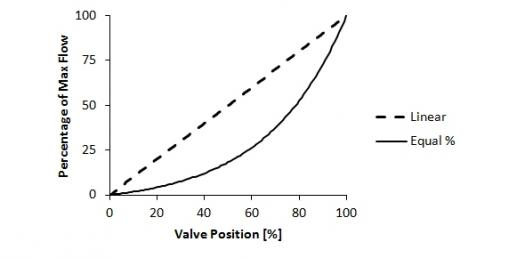# Control Valve Flow Characteristics

5 Questions | Total Attempts: 425Settings.

• 1.
The three most common types of flow characteristics are:
• A.

Linear, Graphical, Random

• B.

Equal Percentage, Un-equal percentage, Quick opening

• C.

Non-linear, Equal percentage, Fast opening

• D.

Linear, Equal percentage, Quick opening

• 2.
What type of characteristic might be best used for liquid level or flow loops?
• A.

Equal Percentage

• B.

Linear

• C.

Quick opening

• 3.
A typical application of an equal percentage characteristic might be:
• A.

Temperature and pressure control

• B.

Liquid level control

• C.

Frequent on/off service

• D.

DeviceNet control

• 4.
The type 86(7)92/86(7)93 features a transfer element which implements 3 different characteristics.
• A.

True

• B.

False

• 5.
It is not possible to customize a characteristic.
• A.

True

• B.

False

Related TopicsBack to top